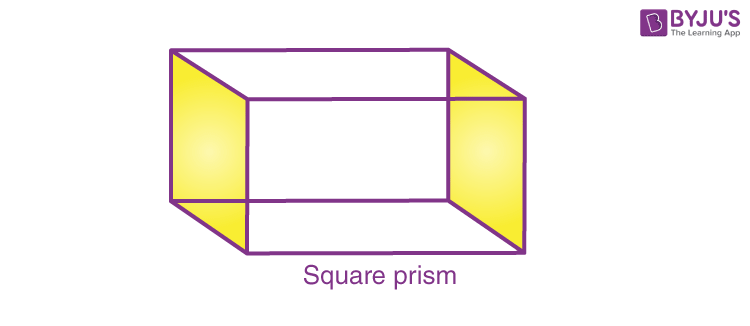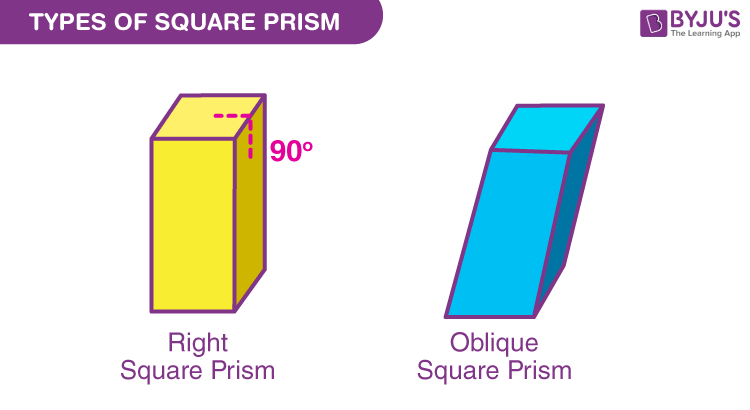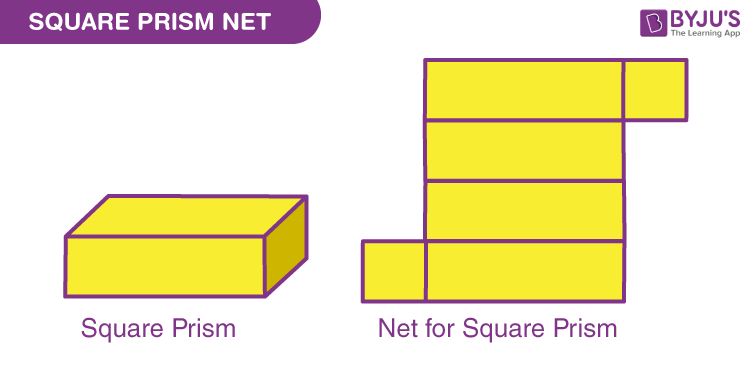Checkout JEE MAINS 2022 Question Paper Analysis : Checkout JEE MAINS 2022 Question Paper Analysis :

# Square prism

A square prism is basically a cuboid, that has square bases. It has four rectangular faces and two square-shaped ends. In Geometry, we have studied various three-dimensional shapes called solid shapes or solids. Some examples are cubes, cuboids, cylinders, prisms, spheres, hemispheres, and so on.

A square prism is another type of 3d shape that is a cuboid, with its two-end faces square in shape. An interesting fact is, all the cubes are square prisms, but not all the square prisms are cubes. It is because the lateral faces of the square prism can be rectangular or square.

In this article, we are going to focus on the square prism definition, types, surface area, and volume formulas, net and examples.

## Square prism Definition

A square prism is a three-dimensional shape cuboid figure whose bases are squares and the other four faces are rectangle in shape. The opposite sides and angles are congruent to each other. An example of a shape of a square prism is a magnet bar.

In the given figure, the bases of the prism are square and lateral faces are rectangular, and therefore, it is called a square prism.## Properties of Square prism

A square prism has four rectangular faces between two square faces. Some important properties of the square prism are:

• A square prism has 6 faces, 12 edges and 8 vertices
• Opposite sides of square prisms are parallel to each other
• Opposite sides of square prisms are congruent to each other
• Opposite angles of square prisms are congruent to each other
• Diagonals of square prism bisect each other
• Consecutive angles of square prisms are supplementary

## Types of Square prism

Square prisms are of two types. They are:

• Right Square Prism
• Oblique Square Prism

### Right Square Prism

A prism with square bases is called a square prism. If the lateral faces of a square prism are perpendicular to its base, then it is referred to as the right square prism

• Vertices of a square prism = 8
• Edges of a square prism = 12
• Faces of a square prism= 6 (including bases)

### Oblique Square Prism

If the lateral faces (rectangular faces) of a square prism are not perpendicular to the square bases, then it is known as an oblique prism.## Square Prism Formulas

Since a square prism is a solid shape, hence, it has two main formulas:

• Surface Area of a square prism
• Volume of a square prism

### Surface Area of a Square Prism

The surface area of a square prism is the measure of the total area of a surface of a three-dimensional solid object. It is expressed in square units. The surface area of a prism is defined as the sum of twice the base area and the lateral surface area of the prism.

The surface area of a square prism formula is given as:

 Surface area of the square prism, SA = 2a2 + 4ah square units

where,

a is the side of the square prism

h is the height of the square prism.

### Volume of a Square prism

The volume of a square prism is a measurement of the occupied units of the solid. The volume of a square prism is the number of units used to fill a cube. It is represented by cubic units. The volume of a square prism formula is as follows,

The volume of a square Prism = Area of base × Height of the prism

If “a” is the side of the base, and “h” is the height of the prism, then:

 Volume a Square Prism, V = a2 h cubic units.

## Square Prism Net

If we open and flatten, we can get the net of the square prism. The net of the square prism will give an accurate view of two square faces and four rectangular faces.## Square Prism Solved Examples

Q.1: What is the surface area of a square prism whose side is 3 cm and height is 5 cm?

Solution:

Given:

Side, a = 3 cm

Height, h = 5 cm

The surface area of the square prism, SA = 2a2 + 4ah square units

Now, substitute the given values

SA = 2(3)2 + 4(3)(5) cm2

SA = 2(9) + 4(15) cm2

SA = 18 + 60 cm2

SA = 78 cm2

Therefore, the surface area of a square prism is 78 cm2

Q.2: What is the volume of a square prism whose side is 3 cm and height is 5 cm?

Solution:

Given:

Side, a = 3 cm

Height, h = 5 cm

The Volume a Square Prism, V = a2 h cubic units.

V = (3)2 (5)

V = 9 (5)

V = 45 cm3

Therefore, the volume of a square prism is 45 cm2.

### Practice Questions on Square Prism

1. If the side length and height of the square prism are 5cm and 12 cm, respectively, find its surface area and volume.
2. The volume of a square prism is 160 cu.cm. What is the length of its sides, if the height of the square prism is 10cm?

## Frequently Asked Questions – FAQs

### What is a square prism?

A square prism is a prism that has its end-faces square in shape and the other four faces are usually rectangular in shape.It a three-dimensional cuboid shape.

### What is the formula for square prism?

A square prism has two main formulas.
Surface area of a square prism = 2a2 + 4ah
Volume of a square prism = a2h
where, a side-length and h is the height of prism.

### Are all the cubes a square prism?

Yes, all the cubes are square prisms, but not all the square prisms are cubes.

### How many faces does a square prism has?

A square prism has 6 faces in total.

### What are the types of square prism?

There are two types of square prime, i.e., right square prism and oblique square prism.

Stay tuned with BYJU’S – The Learning App for interesting Maths-related concepts and download the app to watch personalized videos.

Quiz on Square prism